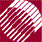ECE532 Biomedical Optics © 1998 Steven L. Jacques, Scott A. Prahl Oregon Graduate Institute

# Time-resolved Monte Carlo

## User settings of variables

The user can set the following variables.

• Number of photons (Nphotons)
• Optical properties (mus, g, nt)
• Spatial bin parameters (NR, radial_size)
• Time point parameters (NTpts, T, T, ..., T[NTpts-1])

The array T[0..NTpts-1] has been declared as T[NPts] in the variables section. In this example, NPTs = 4 and double T was declared. If one wishes to change the number of timepoints being considered, one must manually change the declaration of T to a new T[NPts], as well as choosing NPts and the values T, ..., T[NPts-1] in this user settings section. This is a "minimal Monte Carlo" program choosing simplicity over end-user convenience.

```/**** INPUT
*   Input the number of photons
*   Input the optical properties
*   Input the bin and array sizes
*   Input the time settings
*****/

Nphotons    = 1E3; /* set number of photons in simulation */
/* Optical properties: */
mus         = 100;  /* cm^-1 */
g           = 0.90;
nt          = 1.33;
/* Spatial bin parameters */
NR          = 100;	 /* set number of bins.  */
radial_size = 6.0;   /* cm, total range over which bins extend */
/* Time point parameters: */
NTpts    = 4;        /* number of timepoints */
T        = 0.050e-9; /* 1st timepoint */
T        = 0.100e-9; /* 2nd timepoint */
T        = 0.500e-9; /* 3rd timepoint */
T        = 1e-9;     /* 4   timepoint */
```

NextMonte Carlo